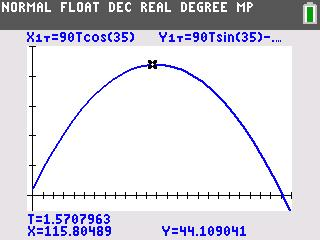# Activities

••• ##### Subject Area

• Math: Precalculus: Parametric Equations

• ##### Author9-12

15 Minutes

• ##### Device
• TI-83 Plus Family
• TI-84 Plus
• TI-84 Plus Silver Edition
•TI-84 Plus C Silver Edition
•TI-84 Plus CE
• ##### Report an Issue

Spring Training#### Activity Overview

Students will explore parametric equations by finding the horizontal and vertical distances traveled by a projectile.

#### Key Steps

•Students are introduced to the use of parametric equations to model the motion of a baseball.

•Students will use the graph to explore key function values that relate to maximum height reached, horizontal distance traveled, and time of travel.

•Students will use Trace to obtain several points on the graph. They enter the points into the List Editor and perform a quadratic regression to generate a quadratic function that corresponds to the parametric equations.